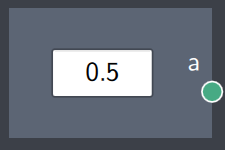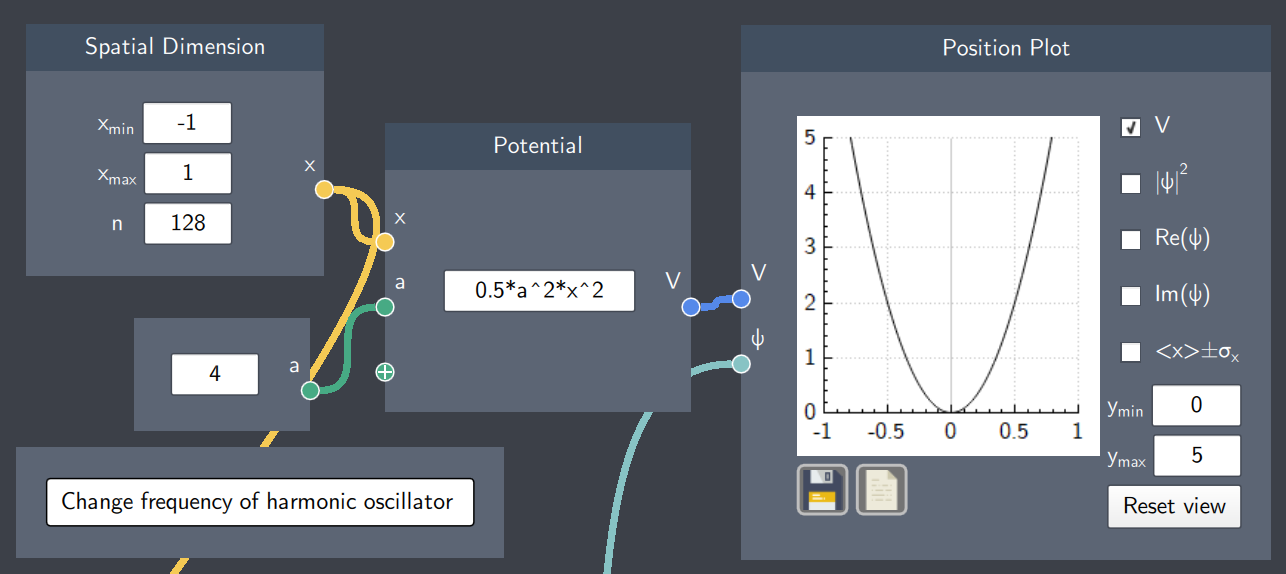# Scalar Input

## Description

With this node, you can input scalar quantities (decimals or integers) to a function.## Input

The node does not require any input from another node.

## Content

The scalar quantity is typed in the content text-field.

## Output

• Scalar (a): The scalar quantity typed in the content text-field.

## Example

In the example below, the scalar input node is used to give a value to the variable 'a' in the Potential node. The potential function here is the harmonic oscillator and the scalar input sets the frequency of the oscillator. Moreover, you can change the frequency of the oscillator by changing the value in the Scalar Input node.